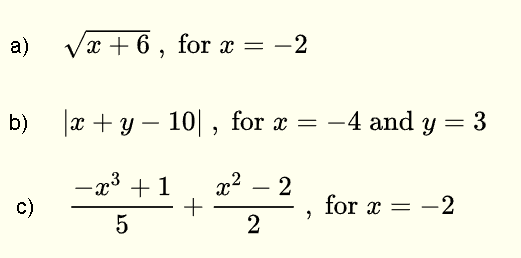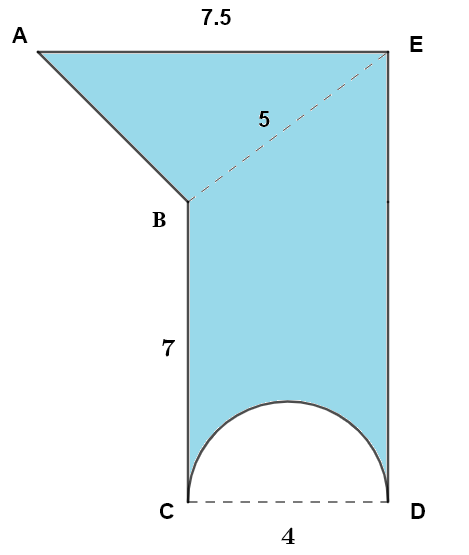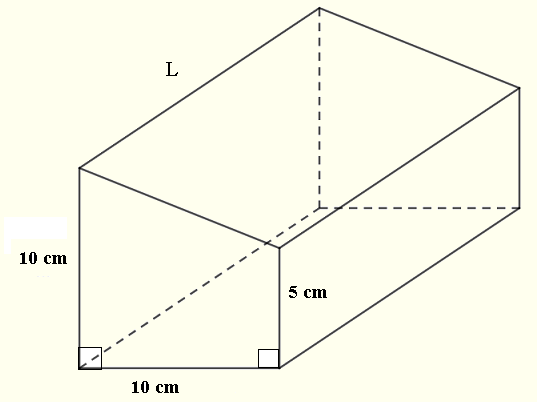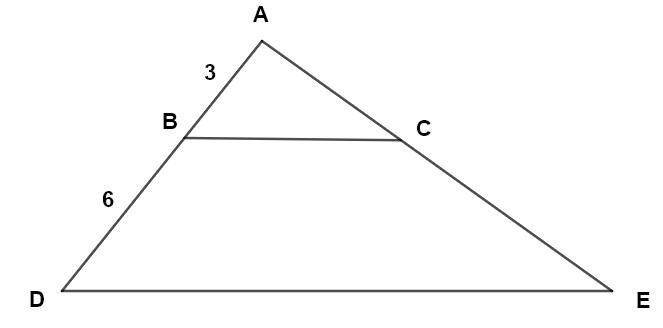# Grade 9 Math Practice Test Questions

Grade 9 math practice test questions on algebra, geometry, trigonometry, and problem solving are presented along with their solutions on videos.

1. Simplify the expressionsSolution on video at Simplify expressions with radicals, question 1

2. Expand and simplify the polynomials belowSolution on video at Expand and simplify polynomials, question 2

3. Simplify the expressions below.Solution on video at Simplify expressions with exponents, question 3

4. Simplify the rational expressionsSolution on video at Simplify rational expressions, question 4

5. Evaluate and simplify the expressions for the given value(s) of the variable(s).Solution on video at Evaluate expressions for given values of the variables, question 5

6. Factor the algebraic expressionsSolution on video at Factor Algebraic Expressions, question 6

7. Solve the following equations.Solution on video at Solve Equations with Fractions, question 7


8. Solve the following equations.
a) $$\quad (x-1)(x+8) = 0$$         b) $$\quad x(x-1) = 1$$         c) $$\quad 2x^2 + 6 x = 8$$
Solution on video at Solve Quadratic Equations, question 8

9. Solve the following equations.
a) $$\quad \sqrt x = 2$$         b) $$\quad \sqrt{x - 2} = 4$$         c) $$\displaystyle \quad \sqrt {\frac{ x}{10}} = 2$$
Solution on video at Solve Equations with Square Roots, question 9

10. Solve the following inequalities and represent the solution set using intervals, graphs on a number line, and inequality symbols.
a) $$\quad 3(x - 2) + 2x \lt -(x+2)$$         b) $$\displaystyle \quad \frac{x + 5}{3} \ge \frac{-x+2}{2}$$         c) $$\displaystyle \quad - (2x - 2) \le 3x + 12$$
Solution on video at Solve Inequalities with Brackets and Fractions , question 10

11. For what value of the parameter $$a$$ is the point with coordinates $$(a,3)$$ on the line whose equation is given by $$2x - 3y = 4$$ ?
Solution on video at Find the x coordinate of a Point on a Line , question 11

12. What is the point of intersection of the lines given by the equations $$2 x + y = 5$$ and $$3x - 2y = 4$$ ?
Solution on video at Find the point of intersection of two lines , question 12

13. What is the slope of each of the lines given by the equations?
a) $$\quad 4x = -2 y + 4$$     b) $$\quad 3y = -9$$     c) $$\quad - 5x = 10$$
Solution on video at Find the slopes of lines , question 13

14. Find the x and y intercepts, if possible, of the lines with equations
a) $$\quad - 3x = 2 y + 6$$     b) $$\quad \displaystyle 2 y = 8$$     c) $$\quad \displaystyle -3x = 6$$
Solution on video at Find the x and y intercepts of lines , question 14

15. a) Find the value of the parameter $$s$$ so that the slope of the line through the points $$(-2 , s)$$ and $$(-4,5)$$ is equal to $$-1$$.
b) Find the equation of the line
Solution on video at Find the y coordinate of a point and the equation of a line , question 15

16. Find the equation of the line through the point $$(-2,4)$$ and perpendicular to the line whose equation is given by $$-2y + 4x = -2$$ ?
Solution on video at find the equation of a line through a point and perpendicular to another line , question 16

17. Find the equation of the line shown below given that the length of segment AB is 3.1 and the length of BC is 6.2 and point $$D$$ is on the line.Solution on video at find the equation of a line from its graph , question 17

18. Which of the following lines whose equations are given below has a negative slope?
a) $$\quad 2x - 2y = 0$$     b) $$3x + 6y = 9$$     c) $$- y = 9$$     d) $$- y = - x + 3$$
Solution on video at find out if the slope of a line is negative , question 18

19. In the figure below, a building has the top of the first floor at point C and the top of the second floor at point D. The angles of elevation from point A, at a distance of $$40$$ meters on the ground, to points C and D are $$14^{\circ}$$ and $$21^{\circ}$$ respectively and point B is at the base of the building. Find the height $$DC$$ from the first to the second floor.Solution on video at Height Between Floors Given Angle of Elevation , question 19

20. The population of a small town increased by $$600$$ in a year. This represents a $$5\%$$ increase over the year before. What was the population of the town the year before the increase?
Solution on video at Absolute increase given percentage increase , question 20

21. Find the area of the shaded shape given that AE is parallel to CD which is the diameter of the semi-circle. BC is parallel to ED which is perpendicular to AE. All lengths in mm.Solution on video at Area of a composite shape , question 21

22. Find the area of a regular hexagon whose perimeter is 18 cm.
Solution on video at Area of a regular hexagon , question 22

23. Find the shaded area enclosed by the quarter of a circle and a square of diagonal 10 mm.Solution on video at Area of Region Between a Square and a Quarter of a Circle - Question 23

24. The volume of the right prism is equal to $$1500 \; cm^3$$.a) Find the length $$L$$ of the prism.
b) Find the total surface area (lateral and bases) of the prism.
c) What is the cost of making the prism with a material that costs $$\ 200$$ per meter squared?
Solution on video at Calculate Surface Area and Cost of Right Prism Given its Volume - Question 24

25. Among 100 students, 70 study math, among which some study physics as well, and 50 study physics, among which some study math as well.
a) How many students are enrolled in both math and physics?
b) How many are enrolled in math only?
c) How many are enrolled in physics only?

26. In the figure below, BC is parallel to DE. Find the area of triangle ADE if the area of triangle ABC is equal to $$30 \; cm^2$$27. One liter of water weighs 1 kilogram and 1 cubic decimeter is equal to 1 liter. What is the weight of water contained in a right rectangular prism container with dimensions of 10 cm, 20 cm, and 200 cm?

28. Linda traveled at an average speed of 65 miles per hour going to a city and at an average speed of 50 miles per hour coming back using the same road. She drove a total of 6 hours away and back.
What is the distance from Linda's house to the city she visited? (round your answer to the nearest mile).

29. Which real number has its square equal to the sum of its half and its third?

30. A total of 1100 students are registered at a school. The ratio of boys to girls in this school is equal to 6:5. What is the ratio of boys to the total number of students?

31. There are 200 balls in a container. The balls have one of three colors: red, blue, and green. The ratio of blue balls to red balls is 3:10, and the ratio of green to blue is 7:3. How many balls of each color are there?